# 贯穿 C++ 11 与 C++ 17 的 Lambda 到底是个什么？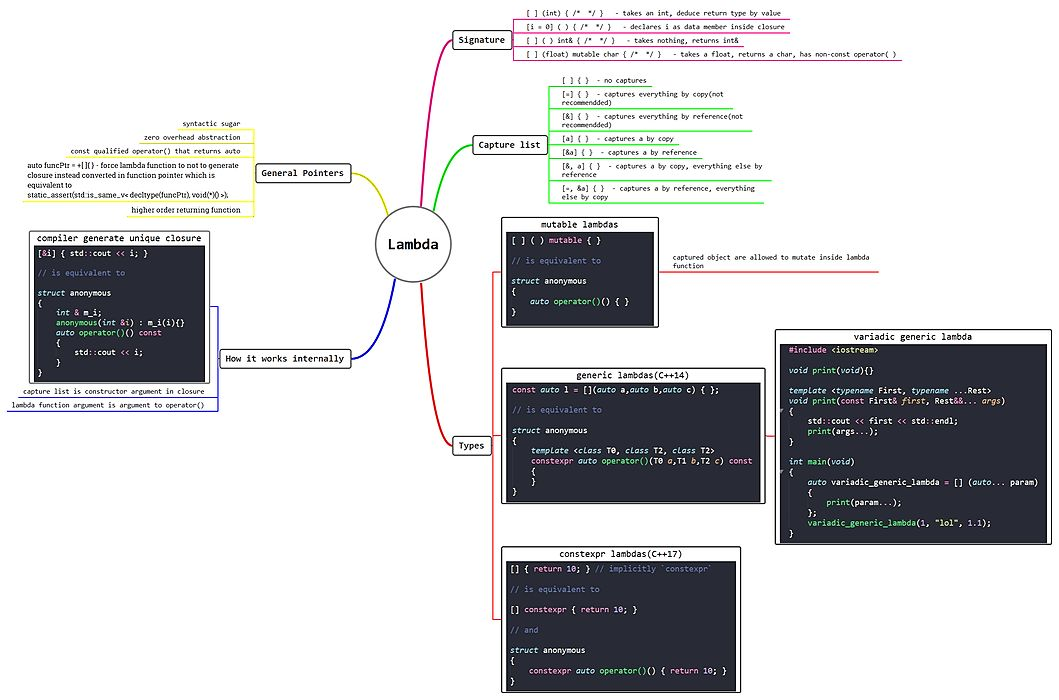Lambda函数是C++ 11中引入的现代C++的一个直观概念，因此在互联网上可以找到大量的关于Lambda函数的文章。但是仍然有一些不为人知的事情（如LIFE-立即调用的函数表达式，Lambda的类型等等）鲜有人谈论。因此，在这篇文章里，我不仅要向你展示C++中的Lambda函数，同时还要介绍它的内部工作机制，以及Lambda函数的其他方方面面。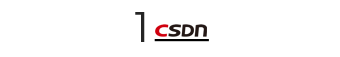Lambda函数是简短的代码片段，它：

[ capture list ] (parameters) -> return-type  {  method definition}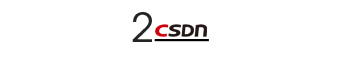C++包含许多有用的通用函数，如std::for_each，它们可以很方便。不幸的是，它们的使用有时也很麻烦，特别是如果你想应用的函子是特定函数的唯一函子的话。以下面的代码为例：

struct print{    void operator()(int element)    {        cout << element << endl;    }};int main(void){    std::vector<int> v = {1, 2, 3, 4, 5};    std::for_each(v.begin(), v.end(), print());    return 0;}

std:for_each(v.begin(), v.end(), [](int element) { cout << element << endl; });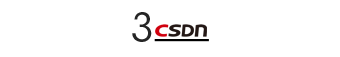Lambda函数内部是如何工作的？

[&i] ( ) { std::cout << i; }// is equivalent tostruct anonymous{    int &m_i;    anonymous(int &i) : m_i(i) {}    inline auto operator()() const    {        std::cout << i;    }};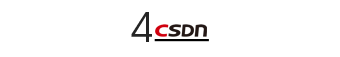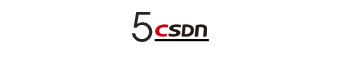int main(){    int x = 100, y = 200;    auto print = [&] { // Capturing object by reference        std::cout << __PRETTY_FUNCTION__ << " : " << x << " , " << y << std::endl;    };    print();    return 0;}

main()::<Lambda()> : 100 , 200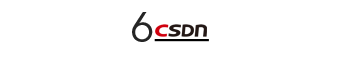Lambda函数的捕获列表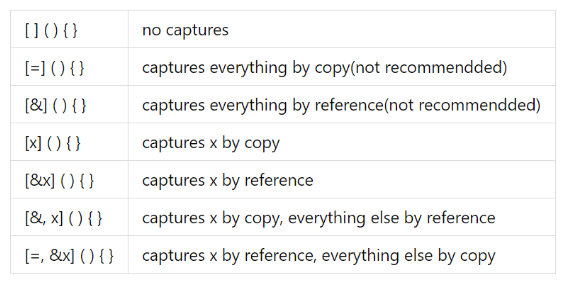template <typename Functor>void f(Functor functor){    std::cout << __PRETTY_FUNCTION__ << std::endl;}/* Or alternatively you can use thisvoid f(std::function<int(int)> functor){    std::cout << __PRETTY_FUNCTION__ << std::endl;} */int g() { static int i = 0; return i++; }int main(){    auto Lambda_func = [i = 0]() mutable { return i++; };    f(Lambda_func); // Pass Lambda    f(g);           // Pass function}

Function Type : void f(Functor) [with Functor = main()::<Lambda(int)>]Function Type : void f(Functor) [with Functor = int (*)(int)]

class Example{public:    Example() : m_var(10) {}    void func()    {        [=]() { std::cout << m_var << std::endl; }(); // IIFE    }private:    int m_var;};int main(){    Example e;    e.func();}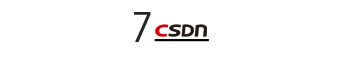C++的Lambda函数类型

const auto l = [](auto a, auto b, auto c) {};// is equivalent tostruct anonymous{    template <class T0, class T1, class T2>    auto operator()(T0 a, T1 b, T2 c) const    {    }};

void print() {}template <typename First, typename... Rest>void print(const First &first, Rest &&... args){    std::cout << first << std::endl;    print(args...);}int main(){    auto variadic_generic_Lambda = [](auto... param) {        print(param...);    };    variadic_generic_Lambda(1, "lol", 1.1);}

[]() mutable {}// is equivalent tostruct anonymous{    auto operator()()  // call operator    {    }};

Lambda作为函数指针，代码如下：

#include <iostream>#include <type_traits>int main(){    auto funcPtr = +[] {};    static_assert(std::is_same<decltype(funcPtr), void (*)()>::value);}

const auto less_than = [](auto x) {    return [x](auto y) {        return y < x;    };};int main(void){    auto less_than_five = less_than(5);    std::cout << less_than_five(3) << std::endl;    std::cout << less_than_five(10) << std::endl;    return 0;}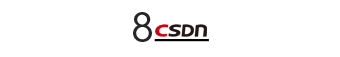constexpr Lambda表达式

constexpr auto sum = [](const auto &a, const auto &b) { return a + b; };/*    is equivalent to    constexpr struct anonymous    {        template <class T1, class T2>        constexpr auto operator()(T1 a, T2 b) const        {            return a + b;        }    };*/constexpr int answer = sum(10, 10);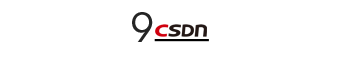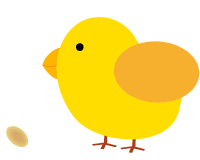点击阅读原文参与开发者大调查，好礼送不停！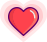09-03391611-1958
12-194209
06-283308
05-29391
03-29770
07-032万+
06-24276
12-21109